Nickzom Calculator Solves Future Value Calculations

According to Investopedia,

Future value (FV) is the value of a current asset at a specified date in the future based on an assumed rate of growth.

If, based on a guaranteed growth rate, a \$10,000 investment made today will be worth \$100,000 in 20 years, then the FV of the \$10,000 investment is \$100,000. The FV equation assumes a constant rate of growth and a single upfront payment left untouched for the duration of the investment.

According to Wikipedia,

Future value is the value of an asset at a specific date. It measures the nominal future sum of money that a given sum of money is “worth” at a specified time in the future assuming a certain interest rate, or more generally, rate of return; it is the present value multiplied by the accumulation function. The value does not include corrections for inflation or other factors that affect the true value of money in the future. This is used in time value of money calculations.

Nickzom Calculator+ (Professional Version) – The Calculator Encyclopedia has a Future Value Calculator this calculator requires certain parameters such as:

• Number of Periods
• Starting Amount
• Interest Rate
• Periodic Contribution
• Contribution at the beginning or end of each payment.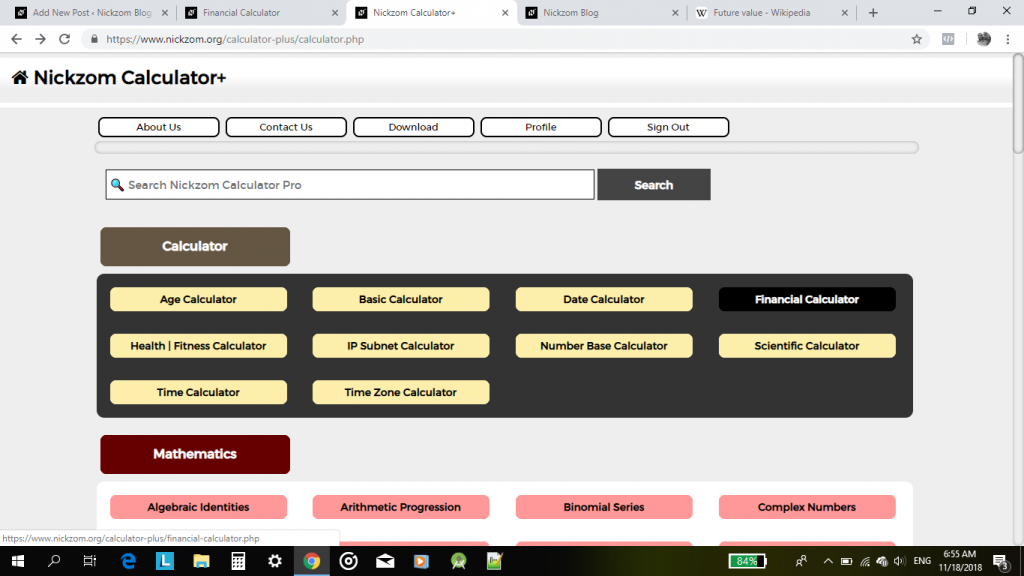The Calculator Encyclopedia Converts Units of Universal Fuel Economy

Nickzom Calculator converts 4 units in fuel economy such as:

• US Miles per gallon
• UK Miles per gallon
• Kilometers per liter
• Liters per 100 Kilometers

According to Wikipedia – Fuel Economy in Automobiles,

The fuel economy of an automobile relates distance traveled by a vehicle and the amount of fuel consumed. Consumption can be expressed in terms of volume of fuel to travel a distance, or the distance travelled per unit volume of fuel consumed. Since fuel consumption of vehicles is a significant factor in air pollution, and since importation of motor fuel can be a large part of a nation’s foreign trade, many countries impose requirements for fuel economy.

For the purpose of this post, let’s convert US Miles per gallon to Kilometers per liter.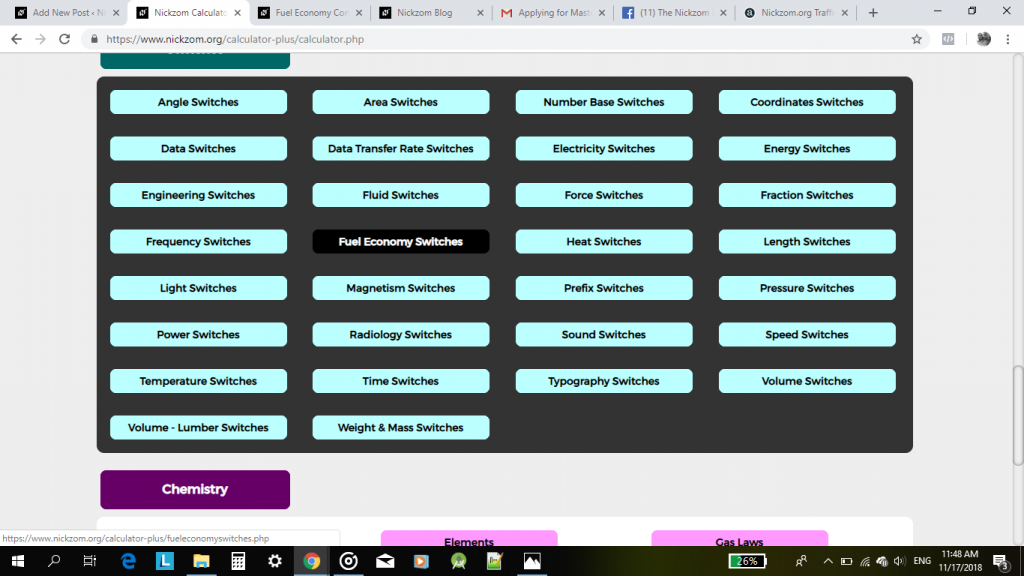Nickzom Calculator Solves Drag Force in Particle Size Analysis for Soil Mechanics

In civil engineering, there are situations where matters of the soil is of utmost importance. Nickzom Calculator solves calculation problems in soil mechanics.

Under Soil Mechanics, there is Soil Test and Particle Size Analysis section.

Today, I would love to introduce you all to Drag Force under Particle Size Analysis.

One can access Nickzom Calculator+ (Professional Version) – The Calculator Encyclopedia via any of these channels:

Parameters to get solution for Drag Force in Particle Size Analysis

• Viscosity
• Radius of Sphere
• Velocity

Nickzom Calculator+ (Professional Version) – The Calculator Encyclopedia solves this problems and shows the steps.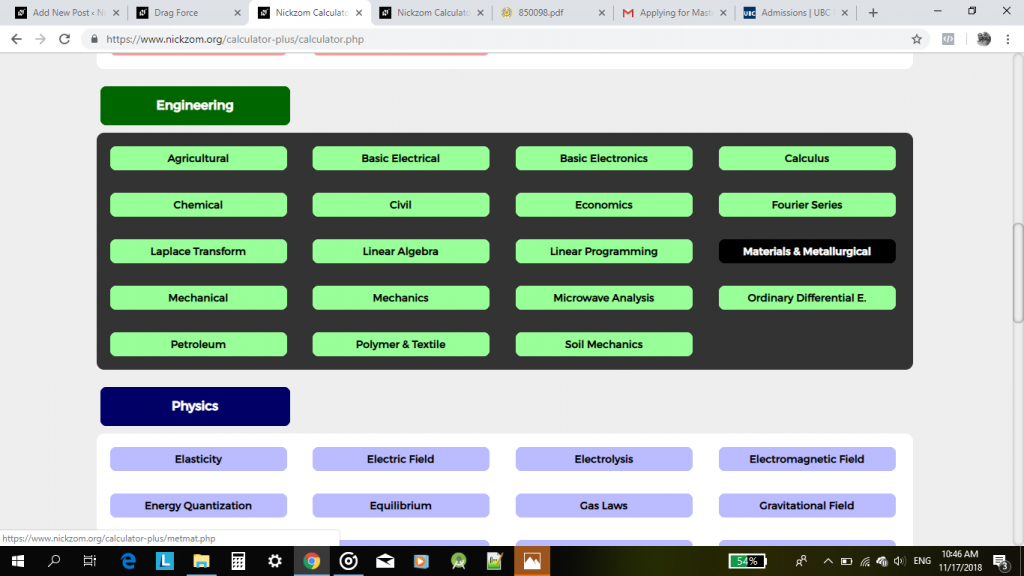Now, click on Soil MechanicsThen, click on Particle Size Analysis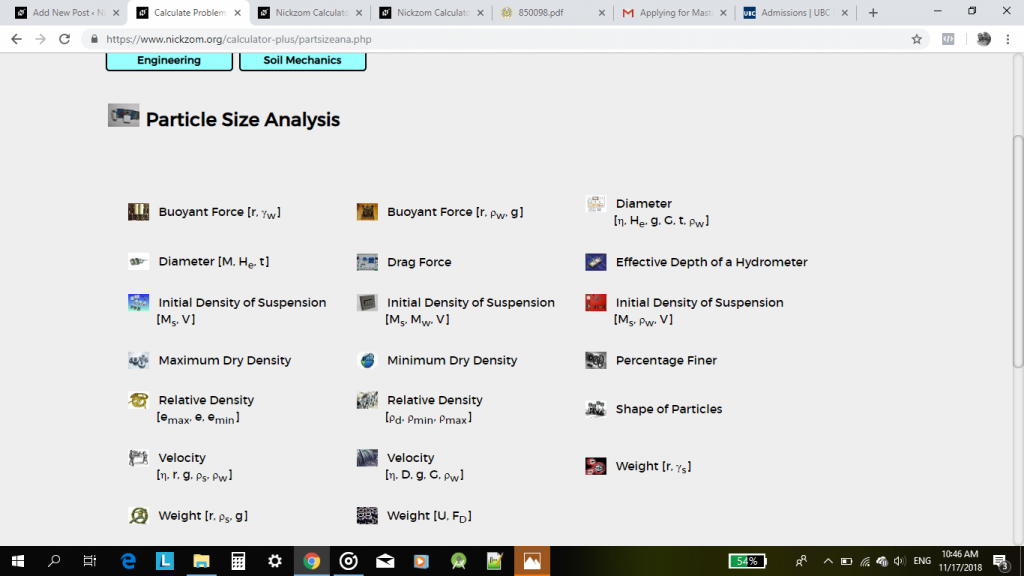Now Click on Drag ForceEnter the values of the parameters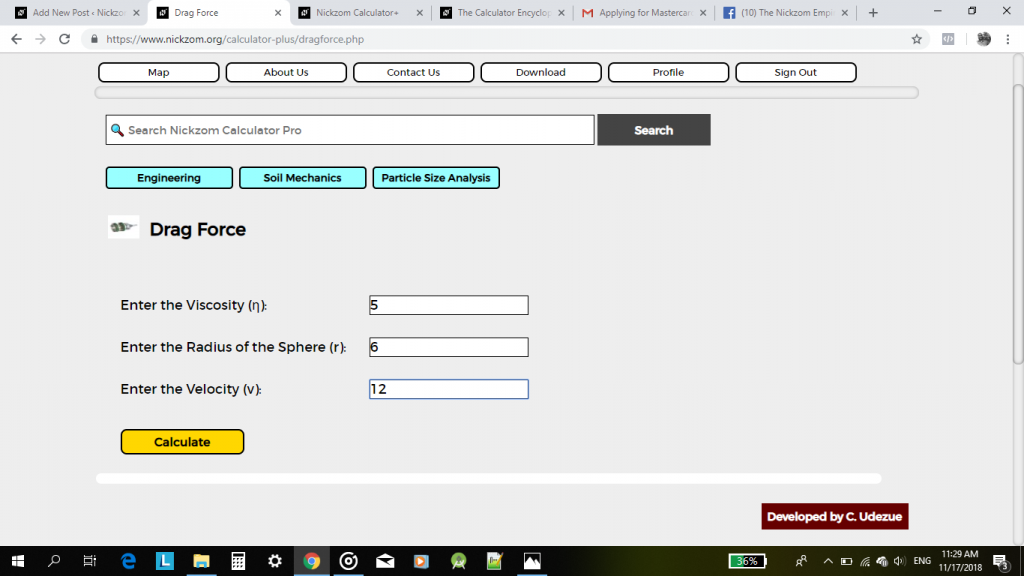Lastly, Click on Calculate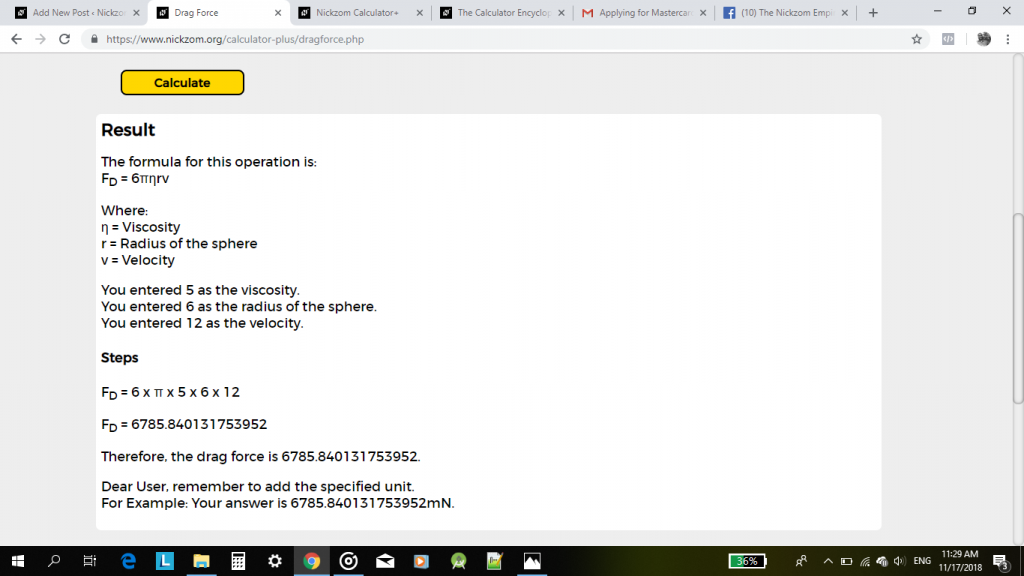Immediately, the answer, formula and workings is displayed for your very own understanding.

The Calculator Encyclopedia now has a Return On Investment (ROI) Calculator

First and Foremost, what is a Return On Investment?

According to Investopedia,

Return on Investment (ROI) is a performance measure used to evaluate the efficiency of an investment or compare the efficiency of a number of different investments. ROI tries to directly measure the amount of return on an particular investment, relative to the investment’s cost. To calculate ROI, the benefit (or return) of an investment is divided by the cost of the investment. The result is expressed as a percentage or a ratio.

According to Wikipedia,

Return on investment (ROI) is a ratio between the net profit and cost of investment resulting from an investment of some resources. A high ROI means the investment’s gains favorably to its cost. As a performance measure, ROI is used to evaluate the efficiency of an investment or to compare the efficiencies of several different investments. In purely economic terms, it is one way of relating profits to capital invested. Return on investment is a performance measure used by businesses to identify the efficiency of an investment or number of different investments.

Return on investment (ROI) measures the gain or loss generated on an investment relative to the amount of money invested. ROI is usually expressed as a percentage and is typically used for personal financial decisions, to compare a company’s profitability or to compare the efficiency of different investments.

Nickzom Calculator+ (Professional Version) – The Calculator Encyclopedia solves ROI calculations and gives one the annualized ROI.

Like every other calculations there are parameters you need to input its values to get a certain result.

The parameters for Nickzom Calculator+ ROI (Return On Investment) are:

• Amount Invested
• Amount Returned
• Investment Length (Number of Years)

One can access Nickzom Calculator+ (Professional Version) – The Calculator Encyclopedia via any of these channels:

Let’s get started,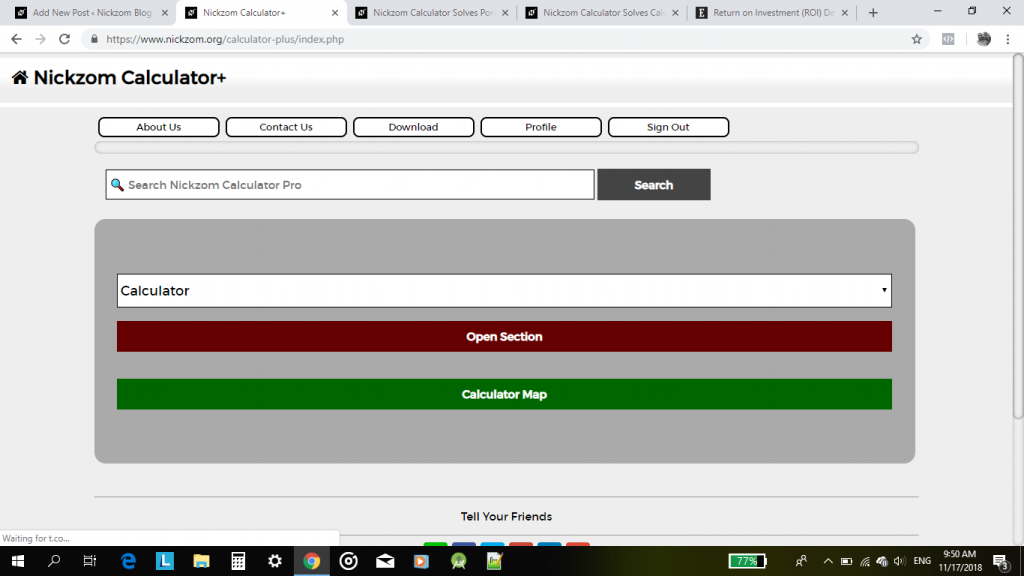First, Click on Calculator Map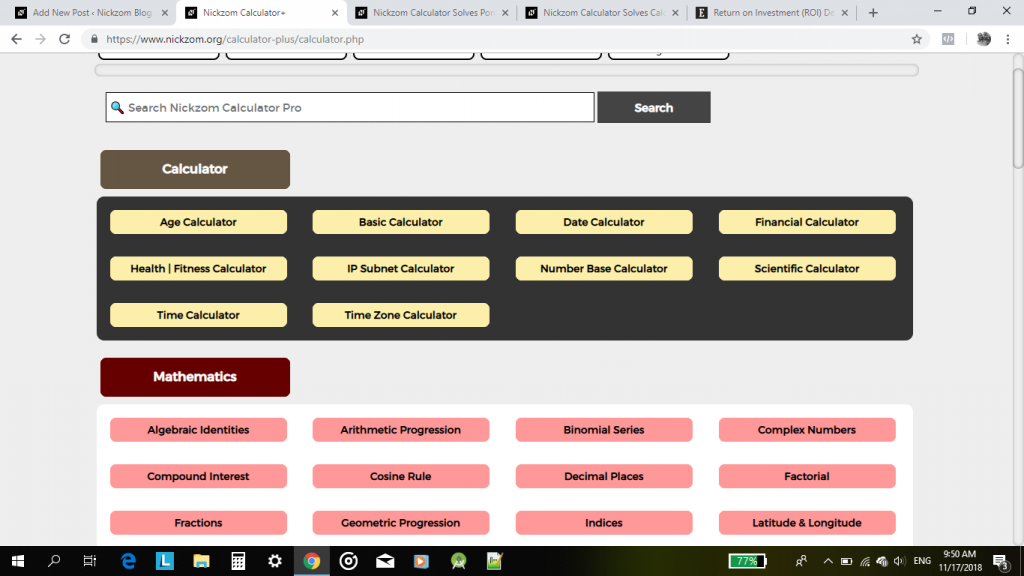Now, click on Financial Calculator,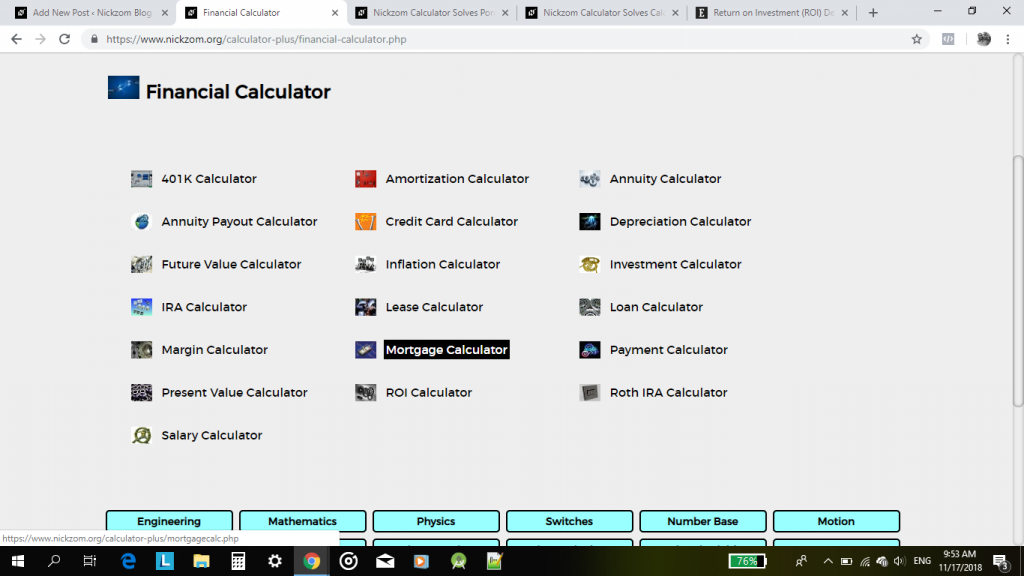Click on ROI CalculatorNow, enter the values of the parameters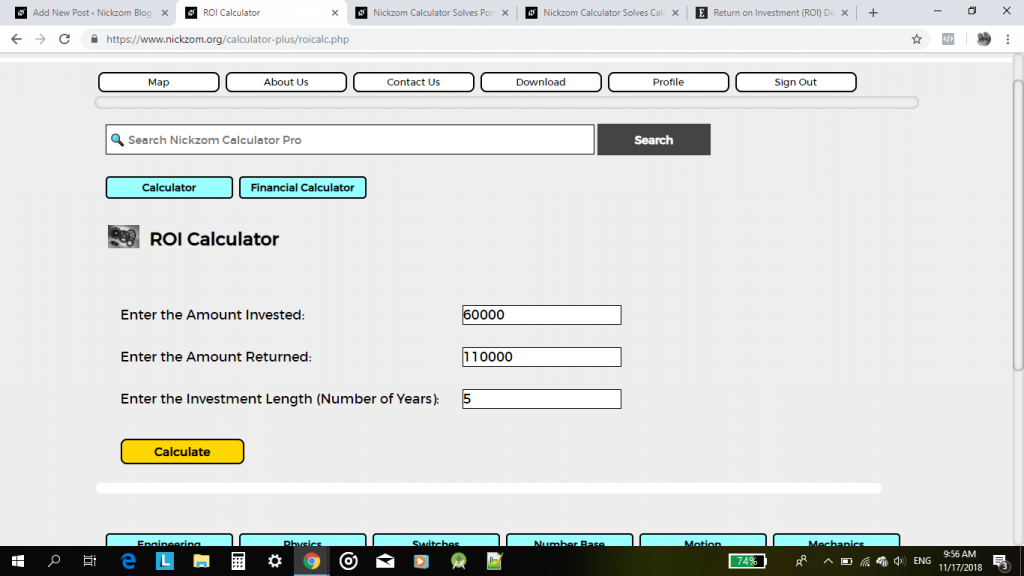Click on Calculate button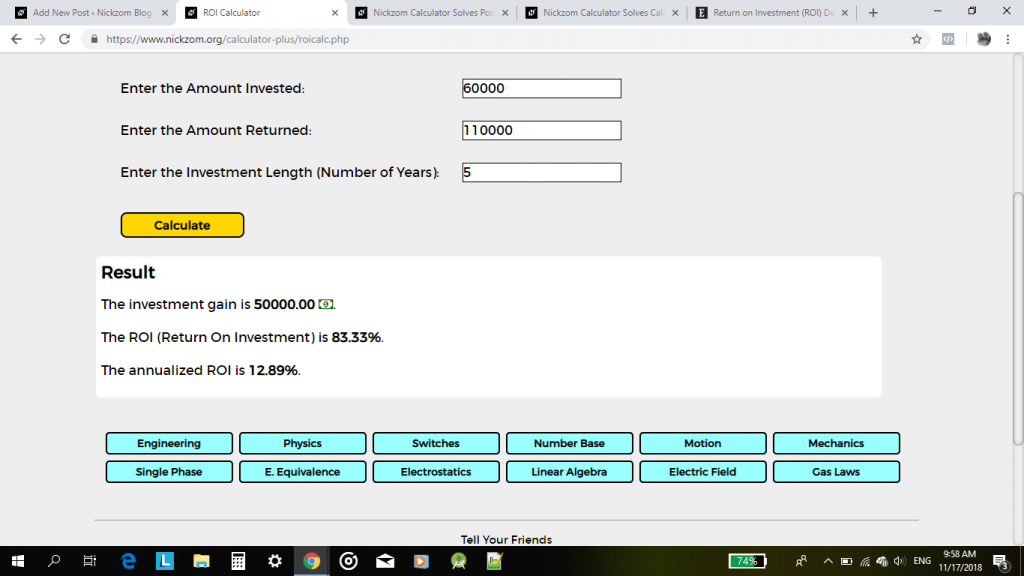There you have it, very simple and easy going with an accurate answer.

Nickzom Calculator Solves Porosity in Sonic Log under Geology – The Calculator Encyclopedia

According to Wikipedia,

Sonic logging is a well logging tool that provides a formation’s interval transit time, designated as, which is a measure of a formation’s capacity to transmit seismic waves. Geologically, this capacity varies with lithology and rock textures, most notably decreasing with an increasing effective porosity

Nickzom Calculator+ (Professional Version) – The Calculator Encyclopedia solves calculations on porosity for sonic log and shows the steps carefully.

The parameters to input to get the answer for your porosity problem are:

• Internal Transit Time of Formation
• Internal Transit Time of Matrix
• Internal Transit Time of the Fluid in the Well Bore
• Compaction Factor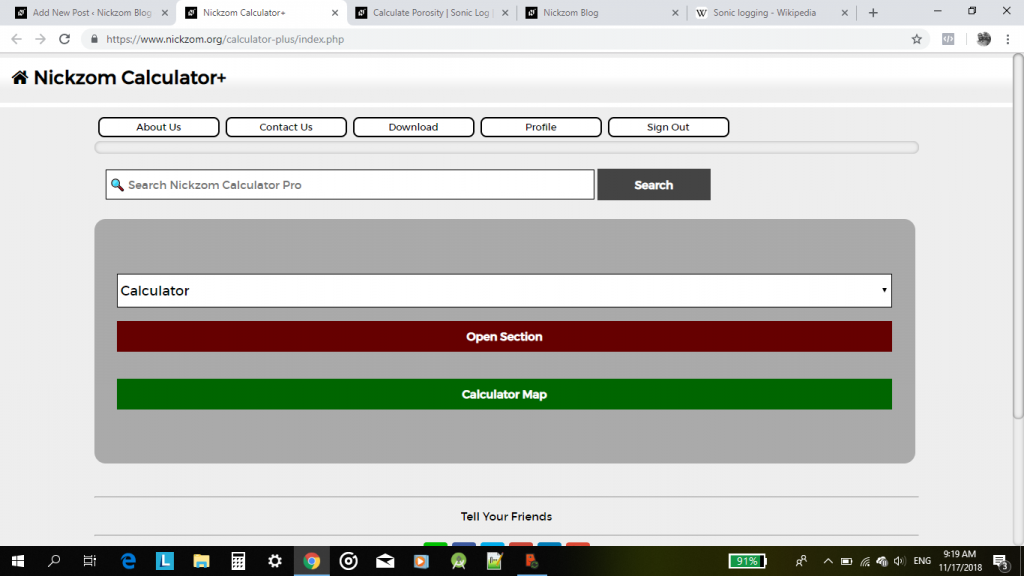Select Geology from the drop down.

Nickzom Calculator Solves Calculations on Electric Field (Physics)

Nickzom Calculator solves for 9 parameters on Electric field such as:

• Heating Effect of Current
• Voltage
• Electric Power
• Capacitance
• Electric Force
• Electric Field Intensity
• Electric Potential Difference
• Electric Work Done
• Energy Stored in a Capacitor

Using Nickzom Calculator to get the value of this parameters is relatively very easy and can be got in a second.

For the purpose of this post, let us find the value of electric field intensity.The Importance and Value of Calculators in SchoolsWith constant advancement in technology there is no doubt, the need to keep up with the dynamics as well as involving technology in every intrinsic part of society. Not doing so can leave a community or nation at large at the mercy of the developed worlds. The simple calculator finds its invention nearly fifty years ago, growing from the simple Abacus, to the basic digital calculator that can perform simple four-function operations (addition, division, multiplication and subtraction) to the more sophisticated machine capable of executing highly technical algebraic symbolic manipulations at high accuracy and speed. The calculator comes in various types, models, sizes and design to fit various budget and user’s preference, very portable and has found its way into various institution including schools.

But what does this mean for learning institutions?

Nickzom Calculator Solves Linear Algebra

Linear Algebra is a matrix based mathematical computation analysis.

According to Wikipedia,

Linear algebra is central to almost all areas of mathematics. For instance, linear algebra is fundamental in modern presentations of geometry, including for defining basic objects such as linesplanes and rotations. Also, functional analysis may be basically viewed as the application of linear algebra to spaces of functions. Linear algebra is also used in most sciences and engineering areas, because it allows modeling many natural phenomena, and efficiently computing with such models. For nonlinear systems, which cannot be modeled with linear algebra, linear algebra is often used as a first-order approximation.

Nickzom Calculator solves 8 major sections of Linear Algebra such as:

• Matrix Arithmetic
• Determinant of a Square Matrix
• Minors and Cofactors of a Square Matrix
• Transpose of a Matrix
• Adjoint of a Square Matrix
• Inverse of a Square Matrix
• Eigenvalues and Eigenvectors of a Square Matrix
• Simultaneous Equation using Inverse MethodUnder, Matrix Arithmetic, there are 6 sub sections which are:

• Addition of Matrices
• Subtraction of Matrices
• Multiplication of a Matrix by a Scalar
• Multiplication of Two Matrices
• Division of a Matrix by a Square Matrix

History and Evolution of Calculators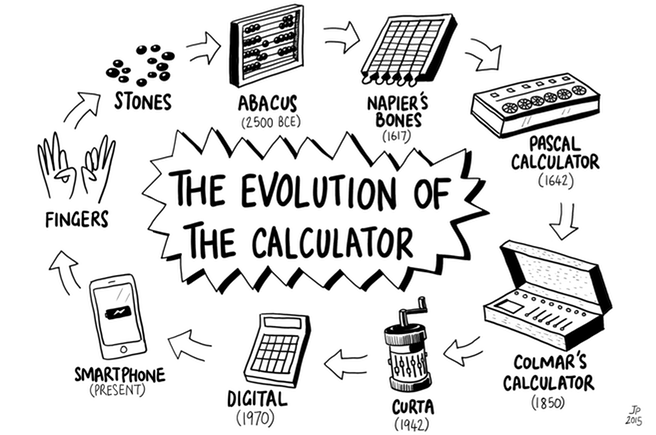How long have we as a species needed to count and calculate numbers? Probably as long as our species have existed. So how did we do it back then? Well, let’s take a rather brief look at how the art of calculating has evolved over the years from fingers and pebbles to advanced computers that can perform complex calculations in split seconds. Fingers and pebbles? Well, that has to be the earliest way anyone ever calculated anything. However, the history of calculators — and by this term I do refer to any equipment or machine that makes it easier and faster to perform rather complex calculations — really begins somewhere around 2,500 to 2,000 BCE with the invention of the Abacus which was first used in Sumeria (now Iraq) and Egypt. It was a wooden frame with slide-able beads on horizontal rods.

Several centuries later, in the 17th century, Napier’s Bones was invented. The bones were thin rods inscribed with multiplication tables and when the vertical alignment of the rods are adjusted, the user can read off his multiplication totals horizontally. Napier’s bones and the abacus remained in accountant’s offices even up to the 19th century, even though other calculating inventions came up. It was in the same 17th century that the slide rule was invented. It consisted of a sliding stick that could perform rapid multiplications by using logarithmic scales and it stuck around even up to the 1980’s as it was a pocket size calculating device. Blaise Pascal also invented his Pascal’s Calculator in 1642. The first of its kind, it was a device that performed all four arithmetic operations without relying on human intelligence. It used geared wheels, was considered truly ingenious but were difficult to produce and so only a few were ever made.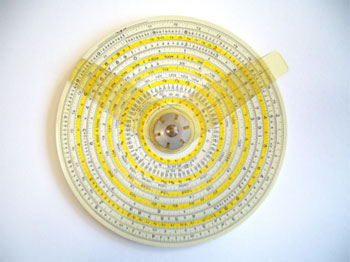Then in 1850, Thomas de Colmar launched the four function Arithmometer, the first mechanical calculator that was considered robust enough for everyday use. His invention was followed by a proliferation of mechanical calculating machines that could perform all four of the arithmetic operations quickly and accurately. The biggest issue with all these devices was that they were usually large and heavy. This problem of size was solved with the invention of the Curta Calculator in 1948 by Curt Herzstark. It was a work of staggering ingenuity at the time, small enough to be held in the hand, able to perform all four arithmetic calculations quickly and accurately and some models could display 15-digit answers (a truly remarkable feet then, considering that an iPhone 6 Plus displays 16-digit answers in scientific mode!). It was the best and the last of the mechanical calculators.As electronics began to take over, several types of calculators were developed starting with ENIAC (Electronic Numeric Integrator And Computer) in 1946, which was a thousand times faster than electro-mechanical computers but used almost 18,000 vacuum tubes, filled a large room and used as much power as a small town. As vacuum tubes gave way to thermionic valves and then to solid state transistors and finally to microchips, electronic calculators continued to be invented from the 1960’s through the 1970’s, each new product trying to better the previous ones in terms of size, power consumption, processing ability and cost. By 1980, pocket calculators had become compact in form, powered by solar cells or button batteries, had a wide variety of functions and were very cheap.

The 1980’s saw the advent of industry-specific calculators which were tailored towards the needs of their respective industries and could perform more advanced and complicated calculations. By the 1990’s, along with the prevalence of PCs and laptops, came the mobile phones which had calculator functions programmed into them. Phones have gotten smarter ever since and calculators have always been a part of mobile phones. On today’s smart phones, one can even get some of those highly specialized types of calculators as just an app in the phone. Despite this proliferation of smartphones with calculators, the ‘dumb’ calculator has not lost its relevance as it is still the choice of people who have to work with a lot of numbers daily and is the only type of computer allowed in most exam halls around the world.

Nickzom Calculator is also one of the best calculators in the world today.

Mathematical Surd Operations can be Solved by Nickzom Calculator – The Calculator Encyclopedia

According to Laerd Mathematics,

Surds are numbers left in root form (√) to express its exact value. It has an infinite number of non-recurring decimals. Therefore, surds are irrational numbers.

According to Math is Fun,

• When it is a root and irrational, it is a surd
• Not all roots are surds

Nickzom Calculator solves calculations on surd and these calculations are categorized into:

• Surd Simplification A
• Surd Simplification B
• ‘Binary’ Surd Addition and Subtraction
• ‘Tenary’ Surd Addition and Subtraction
• Surd Multiplication
• Surd Division
• Surd Expansion
• Surd RationalizationNickzom makes use of a certain procedure to help the user easily input the surd values which is clearly represented in the image below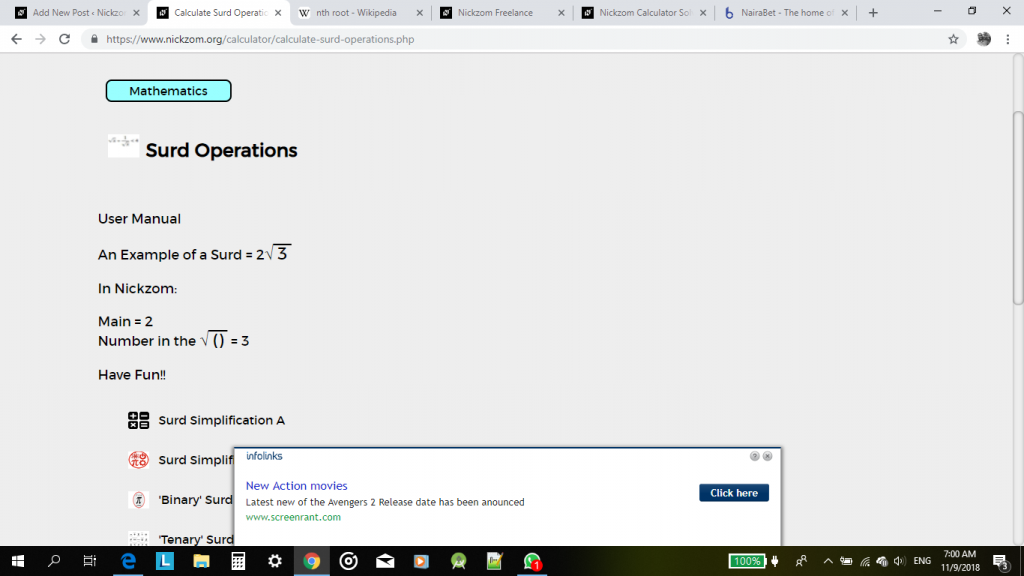For the purpose of this post, we would be handling a step by step guide on how to solve surd rationalization using Nickzom Calculator.

One can access Nickzom Calculator via any of these channels:

Now, click on Surd Rationalization, then enter the values appropriately using the user manual format.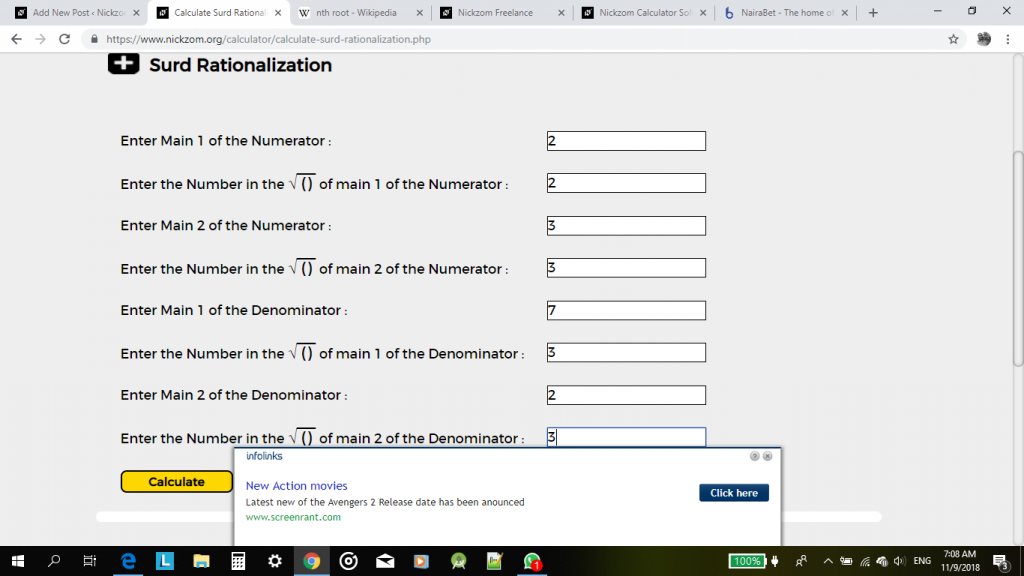Now, click on calculate to display the result.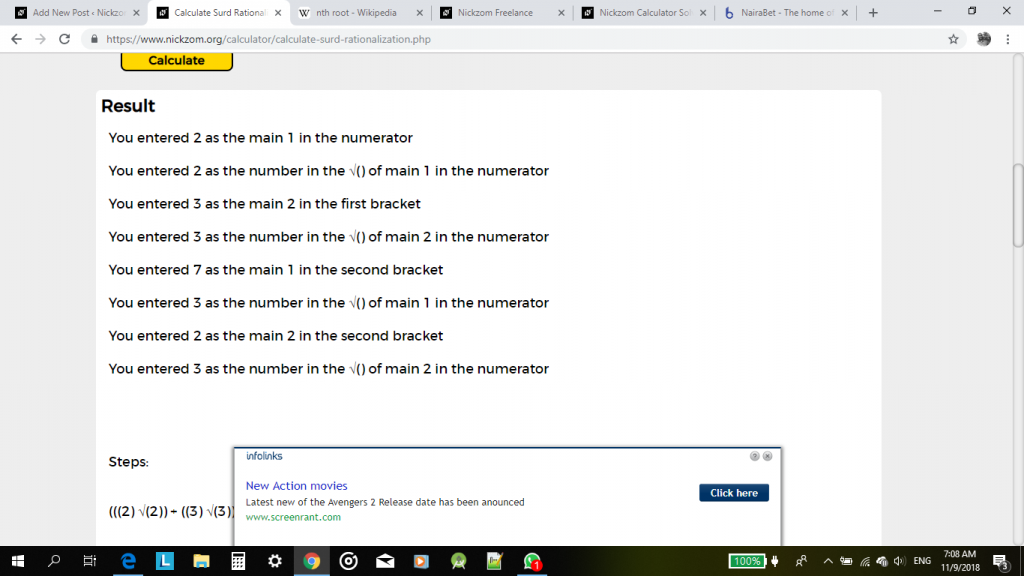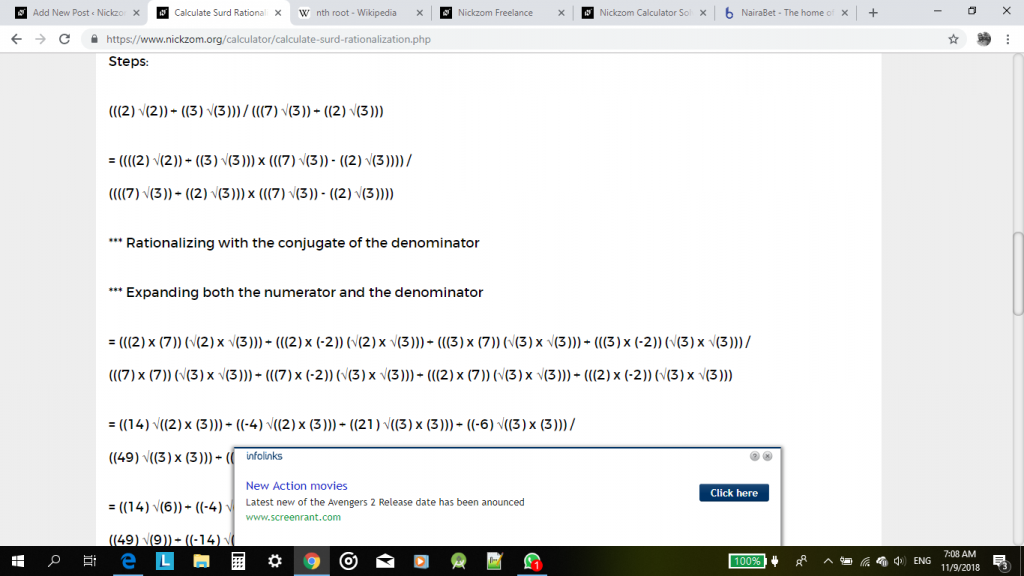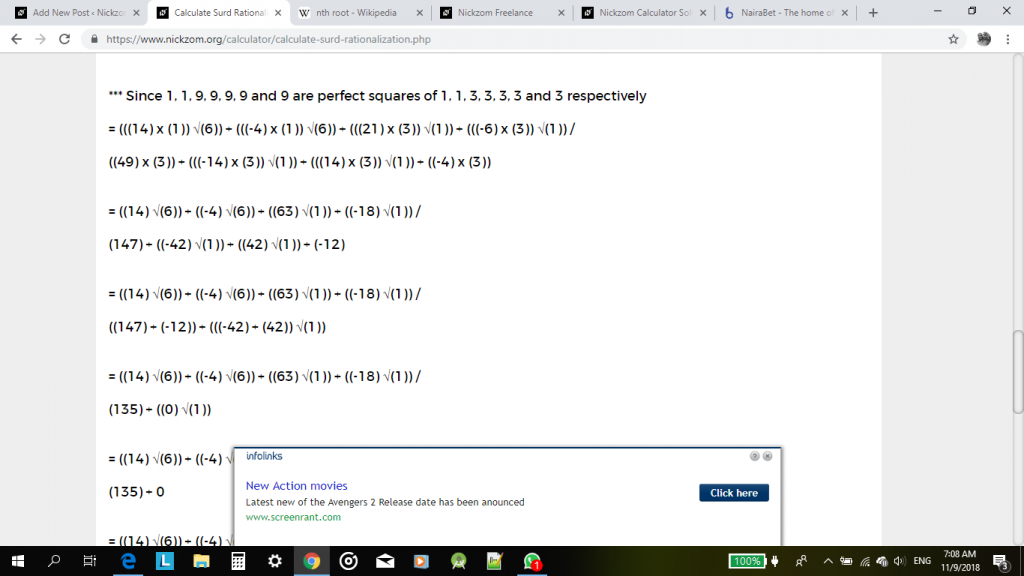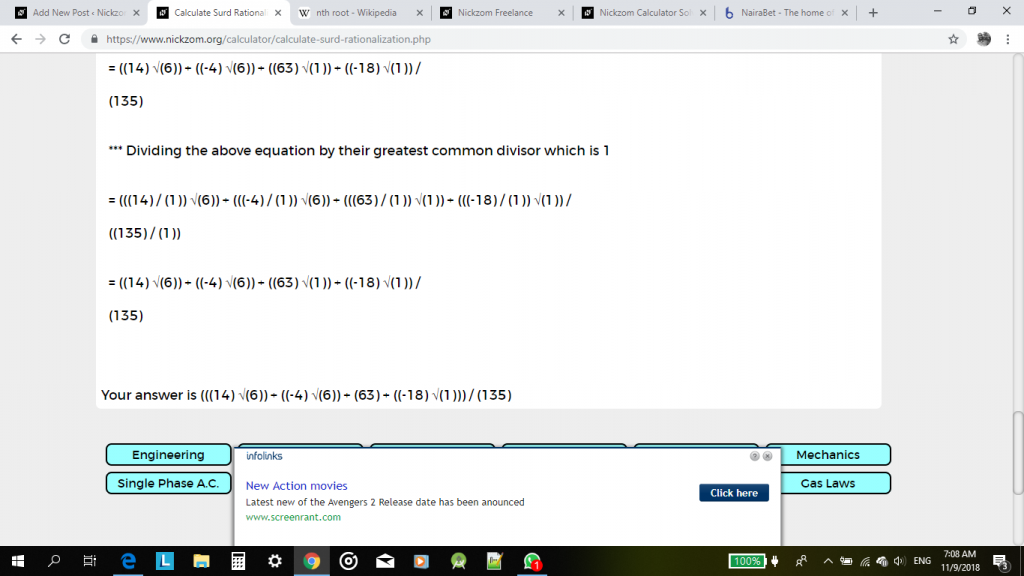I have seen that this steps and procedures might me cumbersome and a bit confusing for a reader or user. Therefore, an update would be coming soon with a new format of solving surd operations that can be easily understood and analysed. For now, with a bit of effort it could be understood or interpreted too.

Watch Out for the Update, Guys!